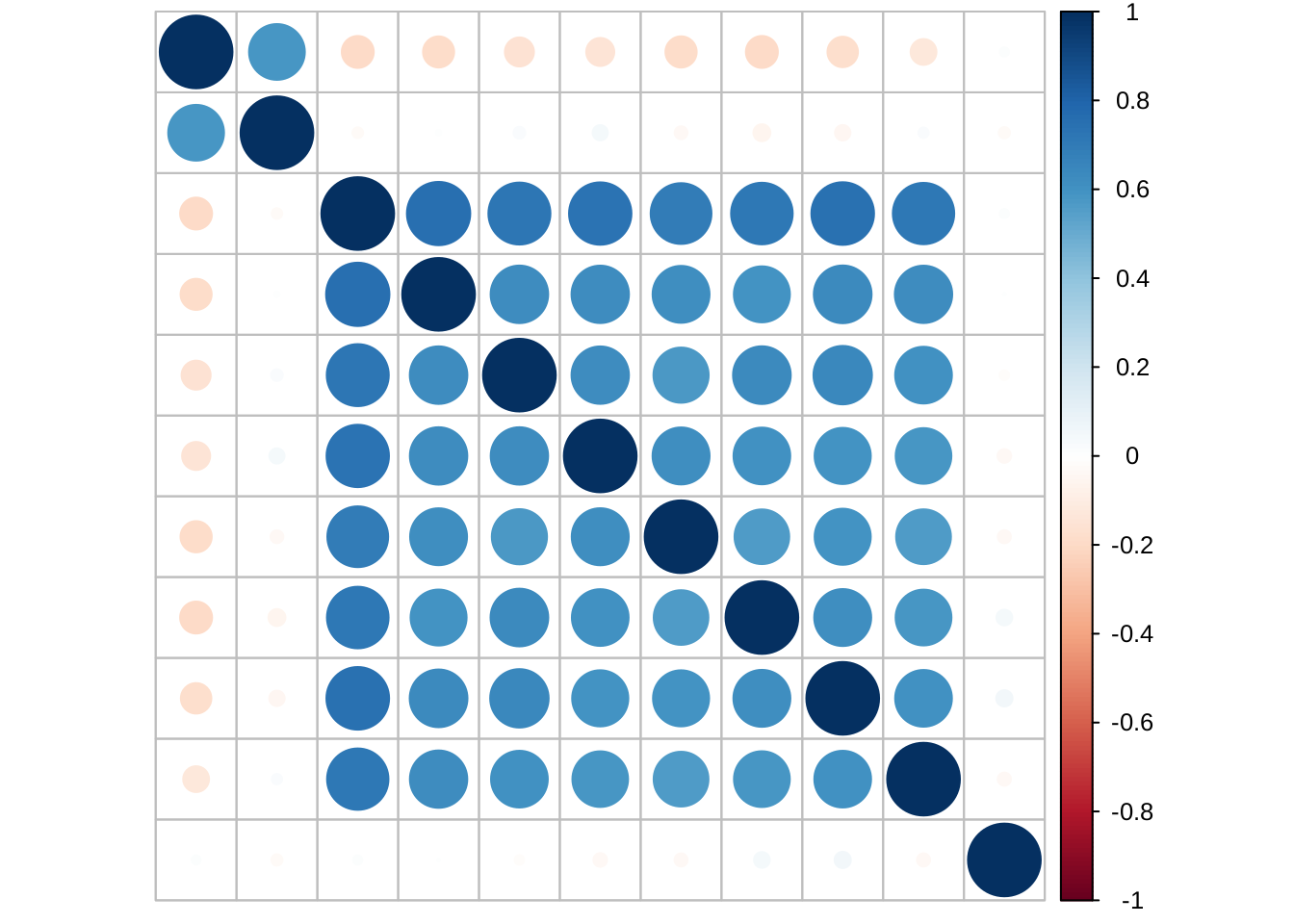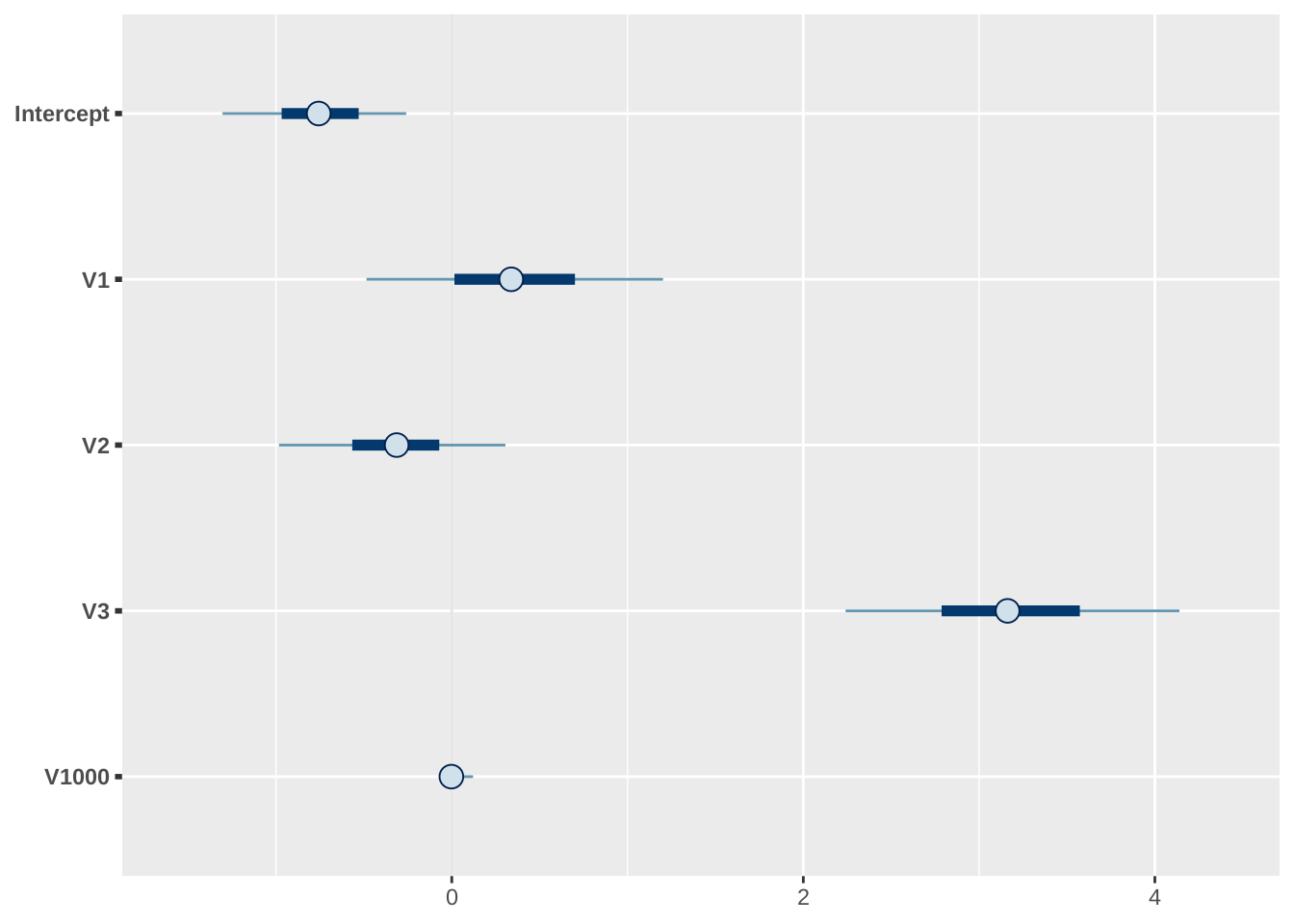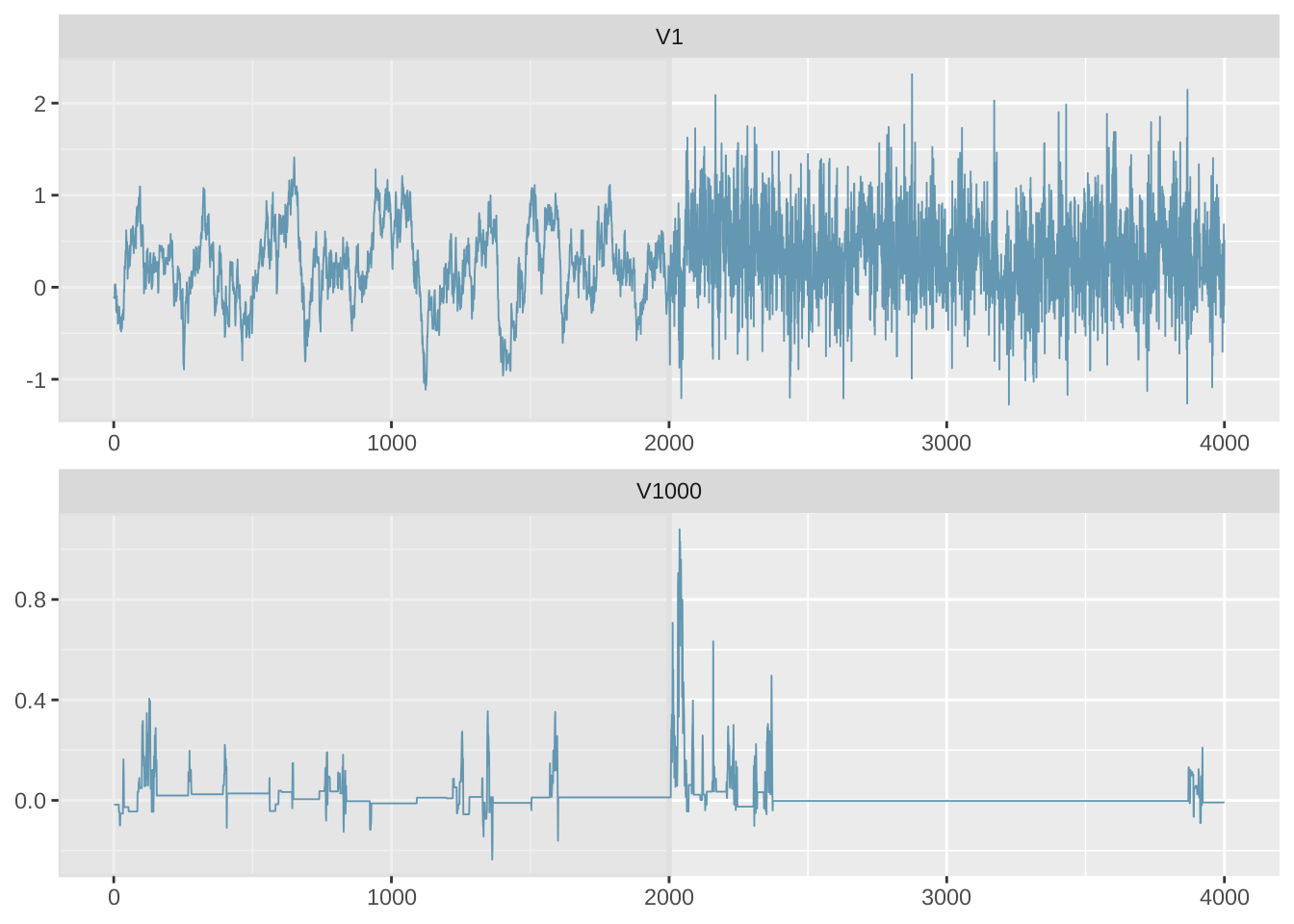# 1 Data Generation

``````library(HTLR)
library(bayesplot)
#> This is bayesplot version 1.7.1
#> - Online documentation and vignettes at mc-stan.org/bayesplot
#> - bayesplot theme set to bayesplot::theme_default()
#>    * Does _not_ affect other ggplot2 plots
#>    * See ?bayesplot_theme_set for details on theme setting``````

The description of the dataset generating scheme is found from Li and Yao (2018).

There are 4 groups of features:

• feature #1: marginally related feature

• feature #2: marginally unrelated feature, but feature #2 is correlated with feature #1

• feature #3 - #10: marginally related features and also internally correlated

• feature #11 - #2000: noise features without relationship with the y

``````SEED <- 1234

n <- 510
p <- 2000

means <- rbind(
c(0, 1, 0),
c(0, 0, 0),
c(0, 0, 1),
c(0, 0, 1),
c(0, 0, 1),
c(0, 0, 1),
c(0, 0, 1),
c(0, 0, 1),
c(0, 0, 1),
c(0, 0, 1)
) * 2

means <- rbind(means, matrix(0, p - 10, 3))

A <- diag(1, p)

A[1:10, 1:3] <-
rbind(
c(1, 0, 0),
c(2, 1, 0),
c(0, 0, 1),
c(0, 0, 1),
c(0, 0, 1),
c(0, 0, 1),
c(0, 0, 1),
c(0, 0, 1),
c(0, 0, 1),
c(0, 0, 1)
)

set.seed(SEED)
dat <- gendata_FAM(n, means, A, sd_g = 0.5, stdx = TRUE)
str(dat)
#> List of 4
#>  \$ X  : num [1:510, 1:2000] -1.423 -0.358 -1.204 -0.556 0.83 ...
#>   ..- attr(*, "dimnames")=List of 2
#>   .. ..\$ : NULL
#>   .. ..\$ : chr [1:2000] "V1" "V2" "V3" "V4" ...
#>  \$ muj: num [1:2000, 1:3] -0.456 0 -0.456 -0.376 -0.376 ...
#>  \$ SGM: num [1:2000, 1:2000] 0.584 0.597 0 0 0 ...
#>  \$ y  : int [1:510] 1 2 3 1 2 3 1 2 3 1 ...``````

Look at the correlation between features:

``````# require(corrplot)
cor(dat\$X[ , 1:11]) %>% corrplot::corrplot(tl.pos = "n")``````Split the data into training and testing sets:

``````set.seed(SEED)
dat <- split_data(dat\$X, dat\$y, n.train = 500)
str(dat)
#> List of 4
#>  \$ x.tr: num [1:500, 1:2000] 0.9157 0.0218 -0.6693 0.4797 0.4862 ...
#>   ..- attr(*, "dimnames")=List of 2
#>   .. ..\$ : NULL
#>   .. ..\$ : chr [1:2000] "V1" "V2" "V3" "V4" ...
#>  \$ y.tr: int [1:500] 1 2 1 2 1 3 2 3 2 3 ...
#>  \$ x.te: num [1:10, 1:2000] 1.031 -0.55 -1.208 -0.858 -1.035 ...
#>   ..- attr(*, "dimnames")=List of 2
#>   .. ..\$ : NULL
#>   .. ..\$ : chr [1:2000] "V1" "V2" "V3" "V4" ...
#>  \$ y.te: int [1:10] 2 1 3 1 3 1 1 1 1 1``````

# 2 Model Fitting

Fit a HTLR model with all default settings:

``````set.seed(SEED)
system.time(
fit.t <- htlr(dat\$x.tr, dat\$y.tr)
)
#>    user  system elapsed
#> 103.543   0.072  18.435
print(fit.t)
#> Fitted HTLR model
#>
#>  Data:
#>
#>   response:  3-class
#>   observations:  500
#>   predictors:    2001 (w/ intercept)
#>   standardised:  TRUE
#>
#>  Model:
#>
#>   prior dist:    t (df = 1, log(w) = -10.0)
#>   init state:    lasso
#>   burn-in:   1000
#>   sample:    1000 (posterior sample size)
#>
#>  Estimates:
#>
#>   model size:    4 (w/ intercept)
#>   coefficients: see help('summary.htlr.fit')``````

With another configuration:

``````set.seed(SEED)
system.time(
fit.t2 <- htlr(X = dat\$x.tr, y = dat\$y.tr,
prior = htlr_prior("t", df = 1, logw = -20, sigmab0 = 1500),
iter = 4000, init = "bcbc", keep.warmup.hist = T)
)
#>    user  system elapsed
#> 180.828   0.363  31.796
print(fit.t2)
#> Fitted HTLR model
#>
#>  Data:
#>
#>   response:  3-class
#>   observations:  500
#>   predictors:    2001 (w/ intercept)
#>   standardised:  TRUE
#>
#>  Model:
#>
#>   prior dist:    t (df = 1, log(w) = -20.0)
#>   init state:    bcbc
#>   burn-in:   2000
#>   sample:    2000 (posterior sample size)
#>
#>  Estimates:
#>
#>   model size:    4 (w/ intercept)
#>   coefficients: see help('summary.htlr.fit')``````

# 3 Model Inspection

Look at the point summaries of posterior of selected parameters:

``````summary(fit.t2, features = c(1:10, 100, 200, 1000, 2000), method = median)
#>                 class 2       class 3
#> Intercept -3.2057501605 -0.7572061810
#> V1        10.7863600649  0.3382765258
#> V2        -6.7701256982 -0.3134671887
#> V3        -0.1955792631  3.1621270554
#> V4        -0.0038819957 -0.0089674488
#> V5         0.0008969358  0.0054764104
#> V6         0.0061773755  0.0797936181
#> V7         0.0140647886  0.0186878519
#> V8         0.0002888387 -0.0023150566
#> V9        -0.0038139108  0.0092575854
#> V10        0.0198427504  0.0153933706
#> V100      -0.0131670180 -0.0039492715
#> V200       0.0069327003 -0.0009540371
#> V1000      0.0080409405 -0.0022844461
#> V2000      0.0014780032  0.0024727814
#> attr(,"stats")
#>  "median"``````

Plot interval estimates from posterior draws using bayesplot:

``````post.t <- as.matrix(fit.t2, k = 2)
## signal parameters
mcmc_intervals(post.t, pars = c("Intercept", "V1", "V2", "V3", "V1000"))``````Trace plot of MCMC draws:

``````as.matrix(fit.t2, k = 2, include.warmup = T) %>%
mcmc_trace(c("V1", "V1000"), facet_args = list("nrow" = 2), n_warmup = 2000)``````The coefficient of unrelated features (noise) are not updated during some iterations due to restricted Gibbs sampling Li and Yao (2018), hence the computational cost is greatly reduced.

# 4 Make Predictions

A glance at the prediction accuracy:

``````y.class <- predict(fit.t, dat\$x.te, type = "class")
y.class
#>       y.pred
#>  [1,]      2
#>  [2,]      3
#>  [3,]      3
#>  [4,]      1
#>  [5,]      3
#>  [6,]      1
#>  [7,]      1
#>  [8,]      1
#>  [9,]      1
#> [10,]      1
print(paste0("prediction accuracy of model 1 = ",
sum(y.class == dat\$y.te) / length(y.class)))
#>  "prediction accuracy of model 1 = 0.9"

y.class2 <- predict(fit.t2, dat\$x.te, type = "class")
print(paste0("prediction accuracy of model 2 = ",
sum(y.class2 == dat\$y.te) / length(y.class)))
#>  "prediction accuracy of model 2 = 0.9"``````

More details about the prediction result:

``````predict(fit.t, dat\$x.te, type = "response") %>%
evaluate_pred(y.true = dat\$y.te)``````